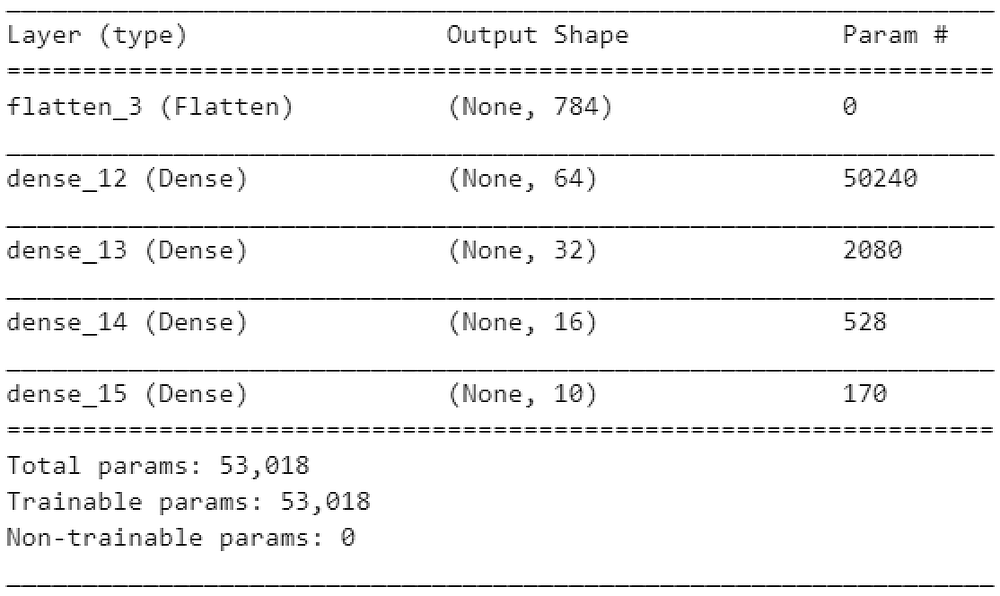# Hyper parameter tuning for Keras models with Scikit-Learn library

Source: Deep Learning on Medium# Hyper parameter tuning for Keras models with Scikit-Learn library

Keras is a neural-network library for the Python programming language capable of running with many deep learning tools such as Theano, R or TensorFlow and allowing fast iteration for experimenting or prototyping neural-networks.

Whether you are prototyping a neural network model in Keras to get a feel for how it will perform the required task or fine tuning a model you have build and tested, there are many parameters to consider for your model. These model parameters are referred to as hyper parameters. The activation function of used in your layers is an example of a hyper parameter. The number of layers in the model, number of neurons per layer or the size of the kernel in a CNN can all be considered hyper parameters.

There is no magic formula to choose the right parameters and different problems will require different approaches. Changing each parameter of your model may affect its performance, and only experimentation will determine which combination works best for your model and data.

In this article we will look at steps required to perform hyper parameter tuning using another machine learning library, Scikit-Learn, to optimize a Keras model. We will build a simple neural network and look for the best optimizer, batch size and the activation using the RandomizedSearchCV object from the Scikit-Learn library.

## Before we begin

The libraries we will be using in our example are TensorFlow, which includes Keras, and Scikit Learn. We will be using the following functions:

`from tensorflow.keras import Sequentialfrom tensorflow.keras.layers import Dense,Flattenfrom tensorflow.keras.datasets import mnistfrom tensorflow.keras.wrappers.scikit_learn import KerasClassifierfrom sklearn.model_selection import RandomizedSearchCV`

We will also use numpy and matplotlib libraries for some support functions:

`import numpy as npimport matplotlib.pyplot as plt`

# Prepare data

To start, lets get a dataset to work with, format it and build our model. Here, we are loading the dataset with a train/test split, normalizing it and printing its shape to ensure we use correct input for the model:

`(X_train, y_trn), (X_test, y_tst) = mnist.load_data()X_trn = X_train[..., np.newaxis].astype(np.float32) / 255.X_tst = X_test[..., np.newaxis].astype(np.float32) / 255.print(X_train.shape,y_trn.shape)print(X_test.shape,y_tst.shape)`